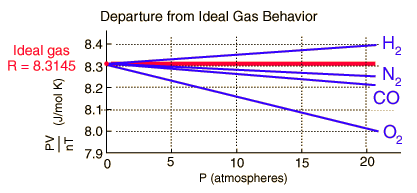Can the ideal gas law be applied to liquids?

Jun 11, 2014

The Ideal Gas Law cannot be applied to liquids.

The Ideal Gas Law is $P V = n R T$.

That implies that $V$ is a variable. But we know that a liquid has a constant volume, so the Ideal Gas Law cannot apply to a liquid.

The Ideal Gas Law doesn't even apply to "real" gases like hydrogen, oxygen, and nitrogen. At ordinary pressures and temperatures, real gases are almost ideal, but they don't obey the Ideal Gas Law perfectly. Real gases always deviate from ideal behaviour.

One way to test the Ideal Gas Law is to rearrange it to get

$R = \frac{P V}{n T}$

and measure the value of $R$ for various gases at different pressures. We should get $R$ = 8.314 kPa·L·K⁻¹mol⁻¹ for all gases at all pressures: the horizontal red line in the diagram below.

Instead, we get the following set of graphs.It all depends on the percentage deviation you are willing to accept before you call the gas non-ideal. Your instructor might accept a deviation of 5 %. A research chemist might not accept a deviation of 0.05 %.